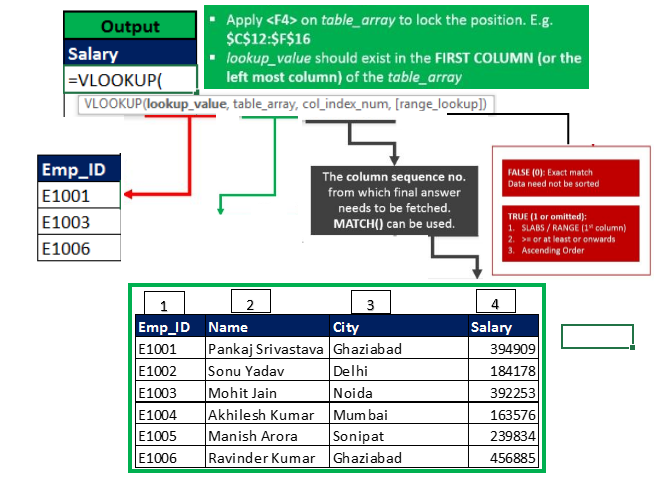# Excel VLOOKUP formula

I am having doubt in Excel formula called VLOOKUP and i tried many times to do it correctly but always i am getting wrong values
kindly help me .

1 Like

Use VLOOKUP when you need to find things in a table or a range by row. For example, look up a price of an automotive part by the part number, or find an employee name based on their employee ID.
In its simplest form, the VLOOKUP function says:

=VLOOKUP(What you want to look up, where you want to look for it, the column number in the range containing the value to return, return an Approximate or Exact match – indicated as 1/TRUE, or 0/FALSE).

Below Two links will surely helps you to solve an issue, just go through it.
https://www.excel-easy.com/examples/vlookup.html
https://exceljet.net/excel-functions/excel-vlookup-function

A common pitfall of VLookup is its inability to look up values left of the lookup_range. Simply put, if the column you have selected to search for reference data (name/index no. etc) lies towards the right of the actual column from which you want to access data from, then the formula doesn’t work.

I may be able to help you out further if you share the exact formulae you are writing along with the dataset.

VLOOKUP- “V” Stand for vertical LOOKUP

If you want to use VLOOKUP formula, you should aware about Vlookup info.

VLOOKUP SYNTAX

=vlookup(lookup_value,table_array_col_index_num,[range_lookup]

lookup_value :- lookup basis of common factor

table_array :- where to look / source data

col_index_no :- which column record want to fetch

[range_lookup] :- 0/False, if you want exact match or 1/True, if you want approximate data